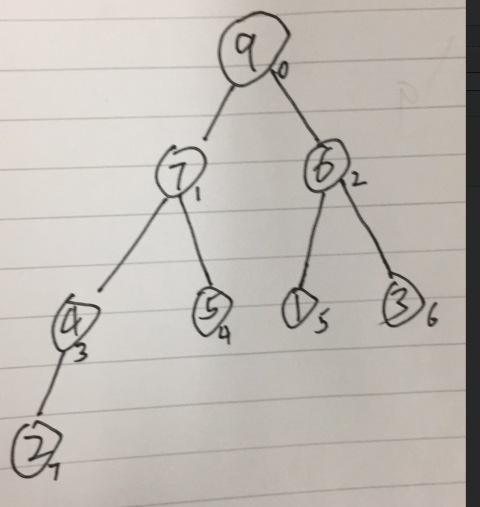堆排序

一：堆结构

即：一个父节点最多只能有两个子节点（可以没有），如下图

二： 数组与堆结构转换

假设已知堆数组   int[]  a = {9,7,6,4,5,1,3,2,}  则相应对结构如下备注： 一个父节点（pNode  为图5中的7 ）和两个子节点(4(lNOde 左节点)和5(rNode 右节点))的关系

左节点：　lNOde =  2*pNode   + 1  ；

右节点 ： 　rNode  = 2*pNode    +2 ；

三：通过已知数组建立成堆结构数组

假设已知数组   int[] a = { 7,9,6,4,5,1,3,2};

java实现：

/**
* 建立堆模型
*
* @param a
*/
private static void buildHeat(int[] a) {
for (int i = 1; i < a.length; i++) {  //注意我是从数组的第二个位置（即 index = 1）开始的
int parentNodeIndex = getParentNodeIndex(i);
int currentIndex = i;
while (parentNodeIndex >= 0) {
// 判断子节点是否大于父节点
if (a[parentNodeIndex] < a[currentIndex]) { // 步骤2  自己大于父节点  交换父节点位置
// 父节点小于子节点 》》交换节点位置
int temp = a[parentNodeIndex];
a[parentNodeIndex] = a[currentIndex];
a[currentIndex] = temp;
} else {
//
break;  //步骤2 ： 自己小于父节点结束循环（while）已成为新的堆结构。>>>>>>>执行步骤3
}
currentIndex = parentNodeIndex;    //步骤2 把自己当成新的子节点
parentNodeIndex = getParentNodeIndex(parentNodeIndex);
}
}
}

四：去堆（获取排序）

步骤一：因为堆顶是整个堆结构中最大的数，所以我获取堆顶的哪个数9（a  如图5）,并把堆中最后一个数2（如图5）放入堆顶 （此时的数组长度是原来数组长度的减一）

步骤二 ：调整堆结构(因为上一个步骤把 最后一个数放入如堆顶).。

调整方法：   获取获取左右子节点，先判断是否有子节点，然后判断两个子节点的大小（假设9已经换成了2，当前它有两个子节点，7(左子节点) 6 （右子节点）），获取到最大的子节点（7），并于父节点（2）比较 ,如果子节点大于父节点，交换父节点与子节点的位置，并把当前自己成为新的父节点重复调整方法，直至没有子节点或父节点大于所有子节点。成为新的堆结构

步骤三 ：重复步骤一和二 ,直至没有改结构中没有子节点。

java实现

备注：代码与步骤二的调整方法有些不同 ,因为 1）如果没有左节点 （调整结束） 就一定不会有右节点？。2）如果有右节点就一定有左节点？。3）如果只有左节点没有右节点？

//去除堆 》》即排序
for(int j = 0; j < a.length; j++) {
int lastIndex = a.length - 1 -j;
int currentIndex = 0;
int temp2 = a;    //步骤1 : 获取堆结构中的最大数  并与最后一个数交换位置
a = a[lastIndex];
a[lastIndex] = temp2;
while(true) {
//获取左节点
int leftChildNodeIndex = getLeftChildNodeIndex(currentIndex);  //步骤2 获取左节点位置
if(leftChildNodeIndex >= lastIndex) {    //判断做节点是否有 有数
//没有左节点子节点 即去堆完成
for (int i = 0; i < a.length ; i++) {
System.out.print(a[i] + ",");
}
System.out.println("没有左节点子节点 即去堆完成");
break; //步骤3
}
//获取右节点
int rightChildNodeIndex = getRightChildNodeIndex(currentIndex);
if(rightChildNodeIndex >= lastIndex) {    //获取右节点
//没有右子节点 但有左节点     需要进行 交换
if (a[leftChildNodeIndex] > a[currentIndex]) {
//判断左子节点  大于父节点  需要交换位置
int temp = a[leftChildNodeIndex];
a[leftChildNodeIndex] = a[currentIndex];
a[currentIndex] = temp;
}
for (int i = 0; i < a.length ; i++) {
System.out.print(a[i] + ",");
}
System.out.println("没有右子节点 但有左节点");
break;   //步骤3
}
//即有左节点又有有节点
//先判断左右节点的大小  返回大节点
int whichIndexBig = a[leftChildNodeIndex] > a[rightChildNodeIndex] ? leftChildNodeIndex : rightChildNodeIndex;
//判断子节点是否大于符节点
if (a[whichIndexBig] > a[currentIndex]) {
//子节点大于父节点  需要交换子父节点的位置
int temp = a[whichIndexBig];
a[whichIndexBig] = a[currentIndex];
a[currentIndex] =  temp;
}
//当前父节点
currentIndex = whichIndexBig;      //
}
}package com.jinliang.sort;

public class HeatSort {
public static void main(String[] args) {
int[] a = { 7,4,6,9,5,1,3,2};
//创建堆数组
buildHeat(a);
for (int j = 0; j < a.length; j++) {
System.out.print(a[j] + ",");
}
System.out.println("建队完成");
//int[] a = {42, 88, 77, 76, 66, 55, 64, 52, 45, 54, 34, 2, 32, 12, 35, 1, 22, 21, 34, 3};
//去除堆 》》即排序
for(int j = 0; j < a.length; j++) {
int lastIndex = a.length - 1 -j;
int currentIndex = 0;
int temp2 = a;
a = a[lastIndex];
a[lastIndex] = temp2;
while(true) {
//获取左节点
int leftChildNodeIndex = getLeftChildNodeIndex(currentIndex);
if(leftChildNodeIndex >= lastIndex) {
//没有左节点子节点 即去堆完成
for (int i = 0; i < a.length ; i++) {
System.out.print(a[i] + ",");
}
System.out.println("没有左节点子节点 即去堆完成");
break;
}
//获取右节点
int rightChildNodeIndex = getRightChildNodeIndex(currentIndex);
if(rightChildNodeIndex >= lastIndex) {
//没有右子节点 但有左节点     需要进行 交换
if (a[leftChildNodeIndex] > a[currentIndex]) {
//判断左子节点  大于父节点  需要交换位置
int temp = a[leftChildNodeIndex];
a[leftChildNodeIndex] = a[currentIndex];
a[currentIndex] = temp;
}
for (int i = 0; i < a.length ; i++) {
System.out.print(a[i] + ",");
}
System.out.println("没有右子节点 但有左节点");
break;
}
//即有左节点又有有节点
//先判断左右节点的大小  返回大节点
int whichIndexBig = a[leftChildNodeIndex] > a[rightChildNodeIndex] ? leftChildNodeIndex : rightChildNodeIndex;
//判断子节点是否大于符节点
if (a[whichIndexBig] > a[currentIndex]) {
//子节点大于父节点  需要交换子父节点的位置
int temp = a[whichIndexBig];
a[whichIndexBig] = a[currentIndex];
a[currentIndex] =  temp;
}
//当前父节点
currentIndex = whichIndexBig;
}
}
//输出排序后的数组
for (int i = 0; i < a.length; i++) {
System.err.print(a[i] + ",");
}
}

/**
* 建立堆模型
*
* @param a
*/
private static void buildHeat(int[] a) {
for (int i = 1; i < a.length; i++) {
int parentNodeIndex = getParentNodeIndex(i);
int currentIndex = i;
while (parentNodeIndex >= 0) {
// 判断子节点是否大于父节点
if (a[parentNodeIndex] < a[currentIndex]) {
// 父节点小于子节点 》》交换节点位置
int temp = a[parentNodeIndex];
a[parentNodeIndex] = a[currentIndex];
a[currentIndex] = temp;
} else {
//
break;
}
currentIndex = parentNodeIndex;
parentNodeIndex = getParentNodeIndex(parentNodeIndex);
}
}
}

// 获取父节点索引
public static int getParentNodeIndex(int currentIndex) {
int parentNodeIndex = (currentIndex - 1) % 2;
Boolean isJO = (parentNodeIndex == 0) ? true : false;
if (isJO) { // 奇数
// 获取父节点
return (currentIndex - 1) / 2;
}
parentNodeIndex = (currentIndex - 2) / 2;
return parentNodeIndex;
}

// 获取left子节点索引
public static int getLeftChildNodeIndex(int currentIndex) {
return 2 * currentIndex + 1;
}
// 获取right子节点索引
public static int getRightChildNodeIndex(int currentIndex) {
return 2 * currentIndex + 2;
}

}
View Code

posted on 2019-02-27 12:50  爱我-中华  阅读(180)  评论(0编辑  收藏  举报